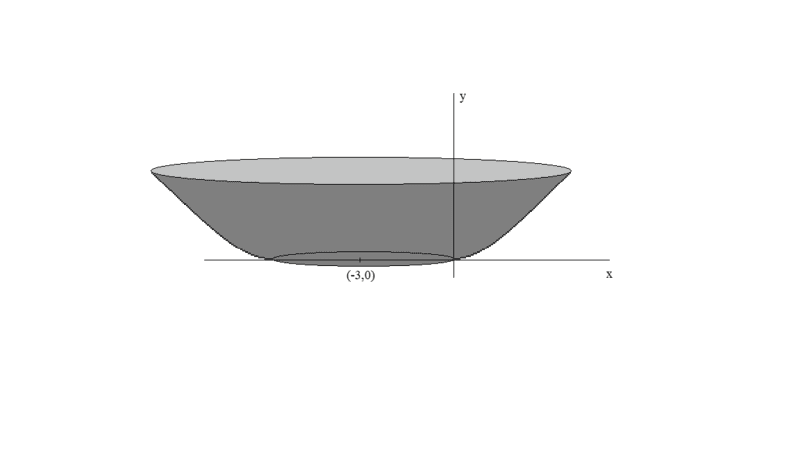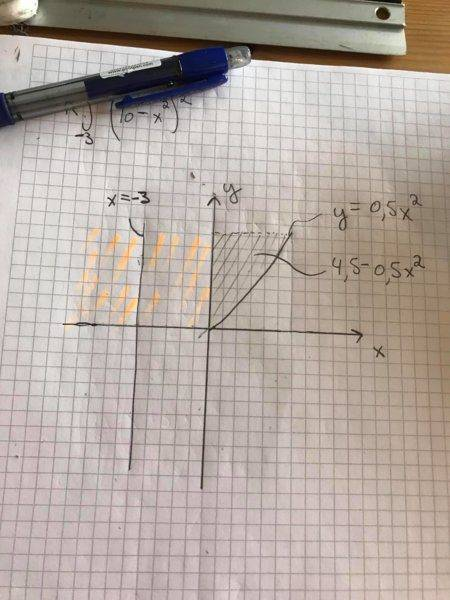# Volume of revolution

## Homework Statement

A container with height $$4.5$$ is created by rotating the curve $$y = 0.5x^2$$ $$0 \leq x \leq 3$$ around $$x = -3$$ and putting a plane bottom in the box. Find the volume $$V$$ of the box.

## The Attempt at a Solution

I want to solve this by using the shell method. I have put up the following integral, which will be the volume of the revolution. It is however not correct, and I haven't really used the information about the height given in the text.. could anyone help me?

$$2\pi\int_0^3 (x+3)(0.5x^2) \, dx$$

Last edited:

mjc123
Homework Helper
You are omitting the central cylinder (between x = 0 and -6).
Personally I think it would be easier to take circular slices in the y direction.

How do you go about calculating the volume of the central cylinder?

Homework Helper
Gold Member
I think you just edited it with the ## 2 \pi ##, and it looks correct to me.

I think you just edited it with the ## 2 \pi ##, and it looks correct to me.
No, the post haven't been edited. I think it´s true what mjc123 said about omitting the central cylinder. I am however not exactly sure how to visualize it.

Homework Helper
Gold Member
No, the post haven't been edited. I think it´s true what mjc123 said about omitting the central cylinder. I am however not exactly sure how to visualize it.
I am a little puzzled by this one also, because it doesn't look like a box. ## \\ ## Edit: I think I see what they are wanting, but I'll let you work on it for a few minutes.

•Kqwert
I am a little puzzled by this one also, because it doesn't look like a box.
I´ve translated it from my own language, so box is probably the wrong description. Let's say container instead. (edited first post)

mjc123
Homework Helper
Oh, and you're also integrating under the curve, i.e. outside the box. Replace 0.5x2 by (4.5 - 0.5x2).
Try drawing a diagram of this to see what the "box" looks like (it's like a bowl).

•mjc123
Homework Helper
This is what I think you're being asked to find the volume of. What is the volume of the bowl?#### Attachments

•Oh, and you're also integrating under the curve, i.e. outside the box. Replace 0.5x2 by (4.5 - 0.5x2).
Try drawing a diagram of this to see what the "box" looks like (it's like a bowl).
Is it like this? We want to find the volume which results when rotating the gray colored area around x = -3, but we also need to include the "inner" volume which I´ve colored orange. The volume of this orange part should be pi*3^2*4.5..?#### Attachments

mjc123
Homework Helper
Looks like you've got it.

Looks like you've got it.
I still get wrong. My answer is (135/2)*pi. Is it the same as you?

mjc123
•